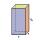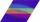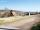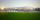Pool

Mr. Peter build a pool shape of a four-sided prism with rhombus base in the garden. Base edge length is 8 m, distance of the opposite walls of the pool is 7 m. Estimated depth is 144 cm.

How many hectoliters of water consume Mr. Peter to fill the pool?

Result

V =  806.4 hl

Solution:Leave us a comment of example and its solution (i.e. if it is still somewhat unclear...):Be the first to comment!To solve this example are needed these knowledge from mathematics:

Do you know the volume and unit volume, and want to convert volume units?

Next similar examples:

1. Water poolWhat water level is in the pool shaped cuboid with bottom dimensions of 25 m and 10 meters, when is 3750hl water in the pool.
2. Swimming pool 4The pool shaped cuboid measuring 12.5 m × 640 cm at the bottom is 960hl water. To what height in meters reaches the water level?
3. Triangular prismCalculate the volume of a triangular prism 10 cm high, the base of which is an equilateral triangle with dimensions a = 5 cm and height va = 4,3 cm
4. Concrete pillarHow many m³ of concrete is needed for the construction of the pillar shape of a regular tetrahedral prism, when a = 60 cm and the height of the pillar is 2 meters?
5. Rhombus HPCalculate area of the rhombus with height 24 dm and perimeter 12 dm.
6. Juice boxThe juice box has a volume of 200ml with its base is an isosceles triangle with sides a = 4,5cm and a height of 3,4cm. How tall is the box?
7. ConvaHow many liters of water fit into the shape of a cylinder with a bottom diameter 20 cm and a height 45 cm?
8. AcreagePlot has a diamond shape, its side is 25.6 m long and the distance of the opposite sides is 22.2 meters. Calculate its acreage.
9. HřištěMap scale is 1: 5000. The playground is rectangular and on the map has dimensions 10 cm and 5 cm What is area of playground in square meters in reality?
10. Rectangular prismIf i have a rectangular prism with a length of 1,000 cm, width of 30 cm and a height of 50 cm, what is the volume?
11. Cylindrical tank 2If a cylindrical tank with volume is used 12320cm raised to the power of 3 and base 28cm is used to store water. How many liters of water can it hold?
12. Concrete boxThe concrete box with walls thick 5 cm has the following external dimensions: length 1.4 m, width 38 cm and height 42 cm. How many liters of soil can fit if I fill it to the brim?
13. Railway wagonThe railway wagon holds 75 m3 load. Wagon can carry a maximum weight of 30 tonnes. What is the maximum density that may have material with which we could fill this whole wagon? b) what amount of peat (density 350 kg/m3) can carry 15 wagons?
14. The shopThe shop has 3 hectoliters of water. How many liter bottles is it?
15. Cuboid aquariumCuboid 25 times 30 cm. How long is third side if cuboid contains 30 liters of water?
16. Liters in cylinderDetermine the height at which level 24 liters of water in a cylindrical container having a bottom diameter 36 cm.
17. Reservoir 3How many cubic feet of water is stored in the reservoir that has a length of 200 feet, a width of 100 feet, an overflow depth of 32 feet, and a current water level of 24 feet?